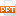# Bài giảng An Introduction to Computer Science Using Java - Chapter 3 Fundamental Data Types of Java

Tài liệu Bài giảng An Introduction to Computer Science Using Java - Chapter 3 Fundamental Data Types of Java: Chapter 3 Fundamental Data Types of JavaLecture Slides to AccompanyAn Introduction to Computer Science Using Java (2nd Edition)byS.N. Kamin, D. Mickunas, E. ReingoldChapter PreviewIn this chapter we will:discuss four important data typesintegersreal numbersstringscharactersdescribe the process of developing and debugging a Java programIntegersNumbers without fractional parts3, 47, -12Variables can be used to store integers using an assignment statementint daysInWeek;daysInWeek = 7;In general integer variables may be used any place an integer literal can beReading Integersimport CSLib.*;Inputbox in;int i;in = newInputBox();in.setPrompt(“Enter an integer: “);i = in.readInt(); Integer Arithmetic OperationsSymbolOperationExample+Addition45 + 5 = 50-Subtraction657 – 57 = 600*Multiplication7000 * 3 = 2100/Division10 / 3 = 3%Remainder10 % 3 = 1Precedence RulesEvaluate all subexpressions in parenthesesEvaluate nested parentheses from the inside outIn the absence of parentheses or within parent...25 trang | Chia sẻ: honghanh66 | Ngày: 19/03/2018 | Lượt xem: 203 | Lượt tải: 0
Bạn đang xem trước 20 trang mẫu tài liệu Bài giảng An Introduction to Computer Science Using Java - Chapter 3 Fundamental Data Types of Java, để tải tài liệu gốc về máy bạn click vào nút DOWNLOAD ở trên
Chapter 3 Fundamental Data Types of JavaLecture Slides to AccompanyAn Introduction to Computer Science Using Java (2nd Edition)byS.N. Kamin, D. Mickunas, E. ReingoldChapter PreviewIn this chapter we will:discuss four important data typesintegersreal numbersstringscharactersdescribe the process of developing and debugging a Java programIntegersNumbers without fractional parts3, 47, -12Variables can be used to store integers using an assignment statementint daysInWeek;daysInWeek = 7;In general integer variables may be used any place an integer literal can beReading Integersimport CSLib.*;Inputbox in;int i;in = newInputBox();in.setPrompt(“Enter an integer: “);i = in.readInt(); Integer Arithmetic OperationsSymbolOperationExample+Addition45 + 5 = 50-Subtraction657 – 57 = 600*Multiplication7000 * 3 = 2100/Division10 / 3 = 3%Remainder10 % 3 = 1Precedence RulesEvaluate all subexpressions in parenthesesEvaluate nested parentheses from the inside outIn the absence of parentheses or within parenthesesEvaluate *, /, or % before + or –Evaluate sequences of *, /, and % operators from left to rightEvaluate sequences of + and – operators from left to rightPrecedence ExamplesExample 16 + 37 % 8 / 5 is the same as6 + ((37 % 8) / 5) = 6 + ( 5 / 5) = 7 Example 26 + 37 % (8 / 5) =6 + 37 % 1 =6 + 0 = 6Additional Integer OperatorsSelf-assignmenttemperature = temperature + 10;Increment cent++; equivalent to cent = cent + 1;Decrement cent--; equivalent to cent = cent - 1;InitializersMay be used to give variables initial valuesint x = 5;int y = 6;Can be written more conciselyint x = 5, y = 6;Can use expressions on the right hand sideint x = 5, y = x + 1;Symbolic ConstantsUseful when you want a variable whose value never changesUse the modifier final in its declaration Examplefinal int US_Population = 278058881;Real NumbersNumbers with fractional parts3.14159, 7.12, 9.0, 0.5e001, -16.3e+002Declared using the type doubledouble pricePerPound = 3.99; taxRate = 0.05; shippingCost = 5.55;The initialization part of the declaration is optionalReal Arithmetic OperationsSymbolOperationExample+Addition4.50e01 + 5.30e00 = 5.03e01-Subtraction6.57e02 – 5.7oe01 = 6.00e02*Multiplication7e02 * 3.0e00 = 2.1e04/Division9.6e01 / 2e01 = 4.8e00Reading Real Numbersimport CSLib.*;Inputbox in;double temp;in = newInputBox();in.setPrompt(“Enter a real number: “);temp = in.readDouble(); StringsString is a class defined in the java.lang packageUnlike other Java classes String has literals and a defined operationExamplesString prompt = “Enter an integer:”;String t1 = “To be “, t2 = “or not to be”;out.print(t1 + t2);Out.print(“Mass is “ + x * 2.2 + “ Kg”);String Method ExamplesOutputBox out = new OutputBox();String s1 = “Here is a test string”;out.println(s1.indexOf(“s”)); // prints 6out.println(s1.indexOf(“x”)); // prints -1out.println(s1.length()); // prints 22out.println(s1.substring(8,14)); // prints ‘a test’Reading Stringsimport CSLib.*;Inputbox in;String input;in = newInputBox();in.setPrompt(“Enter a real number: “);input = in.readString(); CharactersAny key you type on the keyboard generates a character which may or may not be displayed on the screen (e.g. nonprinting characters)Characters are a primitive type in Java and are not equivalent to strings Exampleschar vitamin = ‘’A’, chromosome = ‘’y’, middleInitial = ‘’N’;Important Literal Characters‘A’, ,‘Z’Uppercase letters‘a’, ,‘z’Lowercase letters‘0’, , ‘9’Digits‘.’,’,’,’!’,’”’,etc.Punctuation Marks‘ ’ Blank‘\n’New line‘\t’Tab‘\\’Backslash‘\’’Single Right QuoteCommon Debugging ProblemsMisleading compiler error messagesSyntax errors indicated on one-line may actually reflect an error made on an earlier lineCapitalization errorsJava is case sensitive, identifier names must use the same capitalization rules each timeLogic ErrorsProgram appears to run correctly, but on closer inspection the wrong output is displayedLimitations of Numeric VariablesUnlike the integers mathematics the type int is not infinitely large and it is possible to compute a value incorrectly because the value is too large to be stored in an int variable storage locationUnlike the real numbers in mathematics the type double is not dense, it is not always possible to test double expressions for equality and obtain a correct result due to rounding errors in representationsMixing Numeric Data TypesJava will automatically convert int expressions to double values without loss of informationint i = 5;double x = i + 10.5;To convert double expressions to int requires a typecasting operation and truncation will occuri = (int) (10.3 * x)To round-up instead of truncating add 0.5i = (int) (10.3 * x + 0.5)Mixed Mode Operations and StringsIt is important to remember that “13” and 13 are not the sameExamplesout.println(“4” + “5”) // prints 45out.println(“4” + 5) // prints 45out.println(4 + 5) // prints 9Characters as IntegersIt is legal to assign a char to an int variableint i = ‘a’; // assigns 97 to iIt is legal to assign an int to an char variablechar c = 97; // assigns ‘a’ to cIt is possible to perform arithmetic on char variableschar ch = ‘a’;ch = ch + 1; // assigns ‘b’ to ch

Các file đính kèm theo tài liệu này:

•chapter3_6718.ppt
Tài liệu liên quan